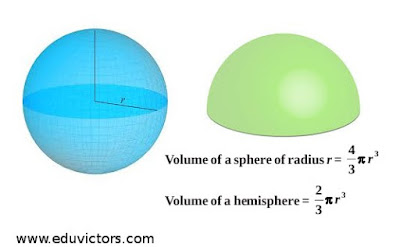## Surface Areas and Volumes - Spheres

Class 9 - Maths - NCERT EXERCISE 13.8

Q1: Find the volume of a sphere whose radius is

(i) 7 cm

(ii) 0.63 m

(i) Given, r = 7 cm
Volume of sphere = (4/3)πr³
= (4/3) × (22/7) × (7)³
= 1437⅓ cm³

(ii) Given,  r = 0.63 m
Volume of sphere = (4/3)πr³
= (4/3) × (22/7) × (0.63)³
= 1.05 m³ (approx)

Q2: Find the amount of water displaced by a solid spherical ball of diameter
(i) 28 cm

(ii) 0.21 m

(i) Given r =28/2 = 14 cm
Volume of water displaced by the spherical ball = (4/3)πr³
= (4/3) × (22/7) × (14)³
= 11498⅔ cm³

(ii) Given r = 0.21/2 = 0.105 m
Volume = (4/3)πr³
= (4/3) × (22/7) × (0.105)³
= 0.004851 m³

Q3: The diameter of a metallic ball is 4.2cm. What is the mass of the ball, if the density of the metal is 8.9 g per cm³?

Answer: Given r = 4.2 / 2 = 2.1 cm

Volume = (4/3)πr³
= (4/3) × (22/7) × (2.1)³ = 38.808 cm³

Density of the metal = 8.9 g/cm³

∴ Mass of the ball = 8.9 × 38.808 g
= 345.39 g (approx)

Q4: The diameter of the moon is approximately one fourth of the diameter of the earth. What fraction of the volume of the earth is the volume of the moon?

Answer: Let diameter of the earth be 2r.
Then radius of the earth = r

Diameter of the moon = 2r/4 =  ½r

∴ Radius of the moon = ¼r

Volume  of the earth = (4/3)πr³

Volume of the moon  = (4/3)π(¼r)³

 Ratio = Volume of the earth = (4/3)πr³ Volume of the moon (4/3)π(¼r)³
⇒ = 64 : 1
⇒ Volume of the earth = 64 times the volume of the moon.

Or Volume of the moon is = (1/64)th of the volume of the earth.

Q5: How many litres of milk can a hemispherical bowl of diameter 10.5 cm hold?

Answer: Given r = 10.5 / 2 = 5.25 cm

Volume of hemisphere bowl = ⅔πr³

= ⅔ × (22/7) × 5.25 × 5.25 × 5.25

= 303 cm³ (approx)
= 303/1000 = 0.303 litres of milk (Answer)

Q6: A hemispherical tank is made up of an iron sheet 1 cm thick. If the inner radius is 1 m, then find the volume of the iron used to make the tank.

Thickness of the iron sheet = 1 cm = 0.01 m

∴ External radius of the tank (R) = 1 + 0.01) m = 1.01 m

Volume of the iron used to make the tank = ⅔π(R³ - r³)

= ⅔ × (22/7) × (1.01³ - 1³)

= ⅔ × (22/7) × 0.030301 = 0.06348 m³

Q7: Find the volume of a sphere whose surface area is 154 cm².

Answer: Given surface area = 154 = 4πr²
⇒ r² = (154  × 7 ) / (4  × 22) = 49/4
⇒ r = 7/2 = 3.5 cm

∴ Volume = (4/3)πr³
= (4/3) × (22/7) × (3.5)³
= 179⅔ cm³

Q8: A dome of a building is in the form of a hemisphere. From inside, it was whitewashed at the cost of Rs 498.96. If the cost of whitewashing is Rs 2.00 per square metre, find the
(i) inside surface area of the dome,
(ii) volume of the air inside the dome.

(i) Inner surface area of the dome = Total cost / Cost of whitewashing per m²

= 498.96 / 2 = 249.48 m²

(ii) Let radius of the dome be r m.
2πr² = 249.48
⇒ 2 × (22/7) × r² = 249.48

⇒ r² = (249.48 × 7) / (2 × 22 ) = 39.69
⇒ r = 6.3 cm

Volume of inner dome = ⅔πr³
= ⅔ × (22/7) × 6.3³
= 523.9 m³

Q9: Twenty seven solid iron spheres, each of radius r and surface area S are melted to form a sphere with surface area S′. Find the
(i) radius r′ of the new sphere.
(ii) ratio of S and S′.

∴ Volume of 27 such spheres = 27 × (4/3)πr³ = 36πr³

Volume of the sphere with radius r′ = (4/3)πr′³
∴ (4/3)πr′³ = 36πr³

⇒ 27r³ = r′³
⇒ r′ = ∛(27r³) = 3r  (Answer)

(ii) Surface area (S) of the sphere with radius r = 4πr²
Surface area (S′) of the sphere with radius r′ = 4πr′²
= 4π(3r)² = 36πr²

 S/S′ = 4πr² 36πr²
= 1/9 = 1: 9 (Answer)

Q10: A capsule of medicine is in the shape of a sphere of diameter 3.5 mm. How much medicine (in mm³) is needed to fill this capsule?

Answer: Given, r = 3.5 / 2 = 1.75mm
Volume of the capsule = (4/3)πr³
= (4/3) × (22/7) × (1.75)³
= 22.46 mm³ (appox.)            ........ Answer